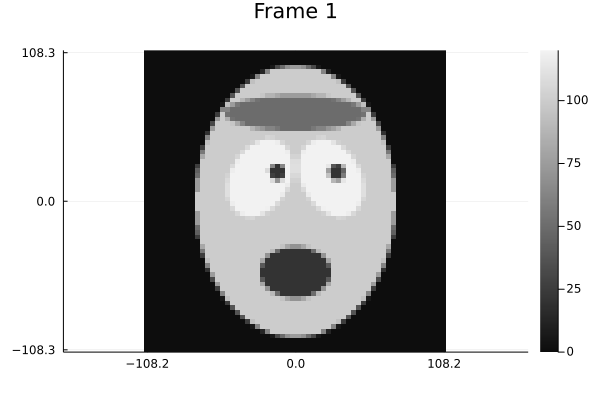# 2D dynamic

This example illustrates 2D dynamic MRI image reconstruction from golden angle (GA) radial sampled k-space data collected with multiple coils (parallel MRI), with temporal "TV" regularizer (corner-rounded) using the Julia language.

This entire page was generated using a single Julia file: 3-2d-t.jl. In any such Julia documentation, you can access the source code using the "Edit on GitHub" link in the top right.

The corresponding notebook can be viewed in nbviewer here: 3-2d-t.ipynb, and opened in binder here: 3-2d-t.ipynb.

using ImageGeoms: ImageGeom
using ImagePhantoms: shepp_logan, SouthPark, phantom, Ellipse
using MIRTjim: jim, prompt
using MIRT: Anufft, diffl_map, ncg
using Plots: gui, plot, scatter, default; default(markerstrokecolor=:auto, label="")
using Plots: gif, @animate, Plots
using LinearAlgebra: norm, dot, Diagonal
using LinearMapsAA: LinearMapAA, block_diag
using Random: seed!
jim(:abswarn, false); # suppress warnings about display of |complex| images

The following line is helpful when running this jl-file as a script; this way it will prompt user to hit a key after each image is displayed.

isinteractive() && jim(:prompt, true);

## Create (synthetic) data

Generate dynamic image sequence:

N = (60,64)
fov = 220
nt = 8 # frames
ig = ImageGeom(; dims = N, deltas=(fov,fov) ./ N)

object0 = shepp_logan(SouthPark(); fovs = (fov,fov))
objects = Array{typeof(object0)}(undef, nt)
xtrue = Array{ComplexF32}(undef, N..., nt)
for it=1:nt
tmp = copy(object0)
width2 = 15 + 5 * sin(2*pi*it/nt) # mouth open/close
mouth = tmp
tmp = Ellipse(mouth.center, (mouth.width, width2), mouth.angle, mouth.value)
objects[it] = tmp
xtrue[:,:,it] = phantom(axes(ig)..., tmp, 4)
end
jimxy = (args...; kwargs...) -> jim(axes(ig)..., args...; kwargs...)
jimxy(xtrue, "True images")

Animate true image:

anim1 = @animate for it in 1:nt
jimxy(xtrue[:,:,it], title="Frame $it") end gif(anim1; fps = 6)Plot one time course to see temporal change: ix,iy = 30,14 plot(1:nt, abs.(xtrue[ix,iy,:]), label="ix=$ix, iy=$iy", marker=:o, xlabel="frame") isinteractive() && jim(:prompt, true); Generate k-space sampling and data: accelerate = 3 nspf = round(Int, maximum(N)/accelerate) # spokes per frame Nro = maximum(N) Nspoke = nspf * nt kspace = ir_mri_kspace_ga_radial(Nro = Nro, Nspoke = Nspoke) fovs = (fov, fov) kspace[:,:,1] ./= fovs kspace[:,:,2] ./= fovs kspace = reshape(kspace, Nro, nspf, nt, 2) (size(kspace), extrema(kspace)) ((64, 21, 8, 2), (-0.0022727272f0, 0.002272619f0)) Plot sampling (in units of cycles/pixel): ps = Array{Any}(undef, nt) for it=1:nt ps[it] = scatter(kspace[:,:,it,1] * fovs, kspace[:,:,it,2] * fovs, xtick=(-1:1)*0.5, ytick=(-1:1)*0.5, xlim=(-1,1).*0.52, ylim=(-1,1).*0.52, aspect_ratio=1, markersize=1, title="Frame$it")
end
plot(ps..., layout=(2,4))